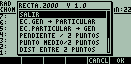## Recta 2000

Description:Asks you for an input (decimals or fractions) and calculates the center of a rectangle, switches from general to particular equation of a rectangle and vice versa, finds the slope of a rectangle, given 2 points, finds the distance between 2 points, finds the shorter distance between a rectangle and a point, finds the equation of a rectangle, given a point and the slope, and finds the equation between 2 points.
Filename:recta2000.zip
ID:4395
Current version:1.0
Author:Dante Camargo
Size on calculator:5 KB
Platforms:49/50
User rating:Not yet rated (you must be logged in to vote)
Primary category:Math/Numeric
Languages:ENG ESP
File date:2000/12/26 14:30:27
Creation date:2000/12/25
Source code:Not included
Screenshot: